Research papers and code for "Chris J. Maddison":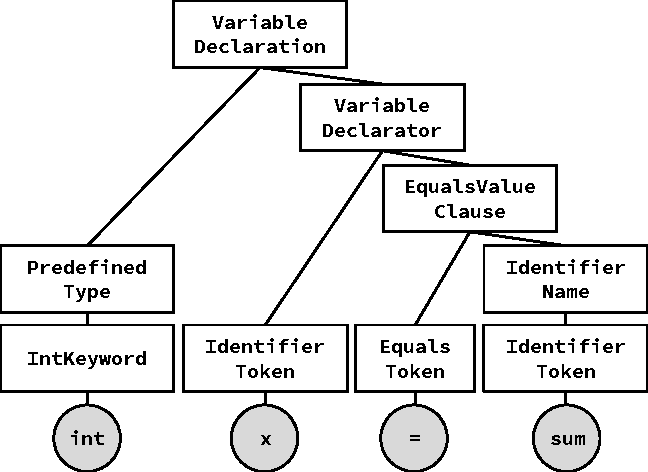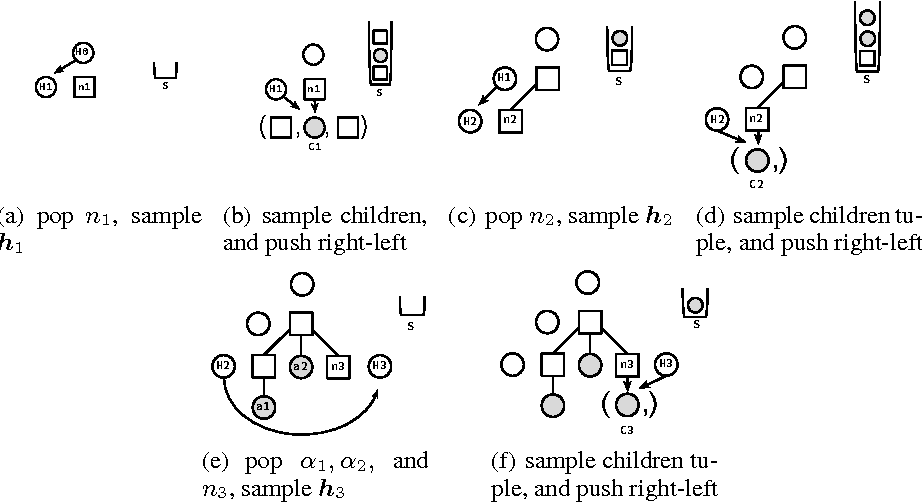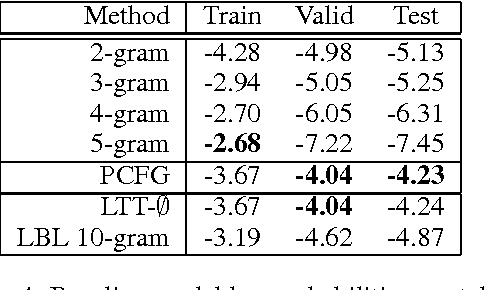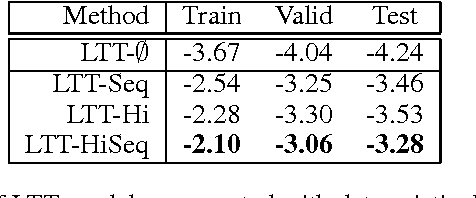We study the problem of building generative models of natural source code (NSC); that is, source code written and understood by humans. Our primary contribution is to describe a family of generative models for NSC that have three key properties: First, they incorporate both sequential and hierarchical structure. Second, we learn a distributed representation of source code elements. Finally, they integrate closely with a compiler, which allows leveraging compiler logic and abstractions when building structure into the model. We also develop an extension that includes more complex structure, refining how the model generates identifier tokens based on what variables are currently in scope. Our models can be learned efficiently, and we show empirically that including appropriate structure greatly improves the models, measured by the probability of generating test programs.

Click to Read Paper and Get Code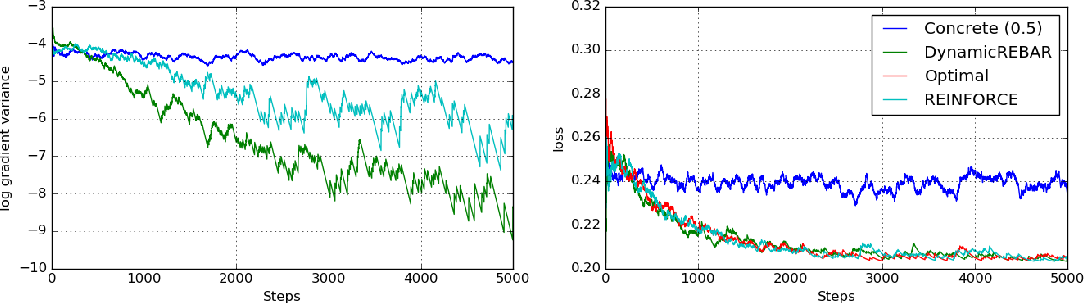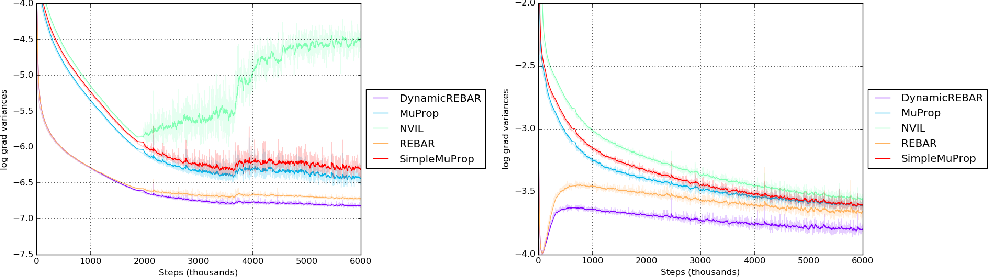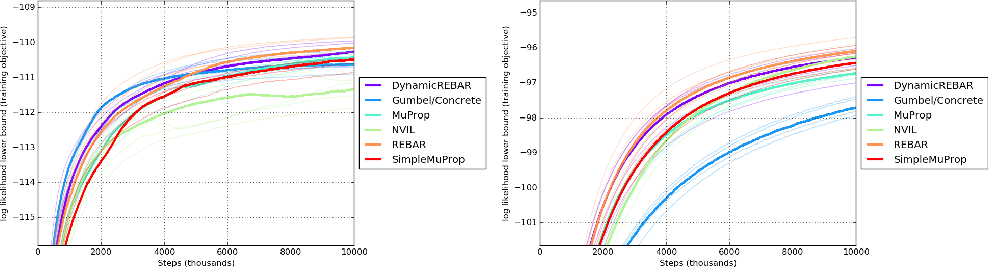Learning in models with discrete latent variables is challenging due to high variance gradient estimators. Generally, approaches have relied on control variates to reduce the variance of the REINFORCE estimator. Recent work (Jang et al. 2016, Maddison et al. 2016) has taken a different approach, introducing a continuous relaxation of discrete variables to produce low-variance, but biased, gradient estimates. In this work, we combine the two approaches through a novel control variate that produces low-variance, \emph{unbiased} gradient estimates. Then, we introduce a modification to the continuous relaxation and show that the tightness of the relaxation can be adapted online, removing it as a hyperparameter. We show state-of-the-art variance reduction on several benchmark generative modeling tasks, generally leading to faster convergence to a better final log-likelihood.

* NIPS 2017
Click to Read Paper and Get Code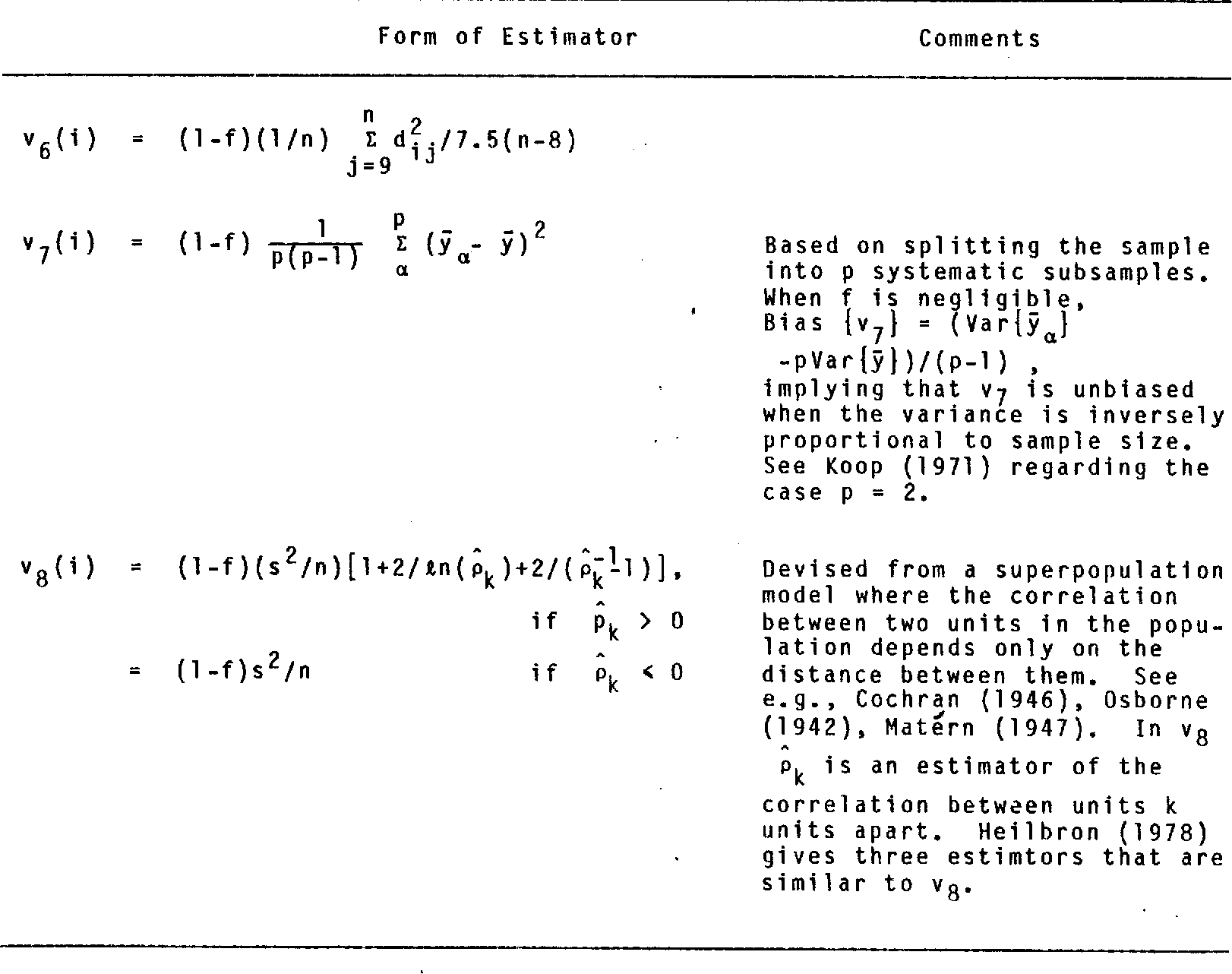The problem of drawing samples from a discrete distribution can be converted into a discrete optimization problem. In this work, we show how sampling from a continuous distribution can be converted into an optimization problem over continuous space. Central to the method is a stochastic process recently described in mathematical statistics that we call the Gumbel process. We present a new construction of the Gumbel process and A* sampling, a practical generic sampling algorithm that searches for the maximum of a Gumbel process using A* search. We analyze the correctness and convergence time of A* sampling and demonstrate empirically that it makes more efficient use of bound and likelihood evaluations than the most closely related adaptive rejection sampling-based algorithms.

* V2: - reworded the last paragraph of Section 2 to clarify that the argmax is a sample from the normalized measure. - fixed notation in Algorithm 1. - fixed a typo in paragraph 2 of Section 5
Click to Read Paper and Get Code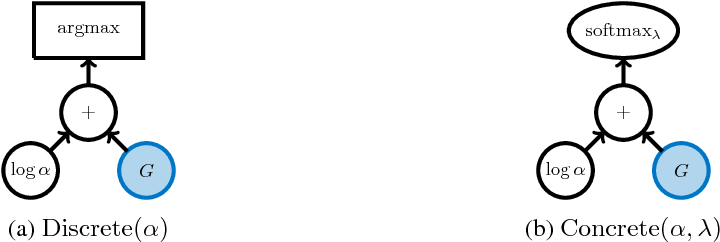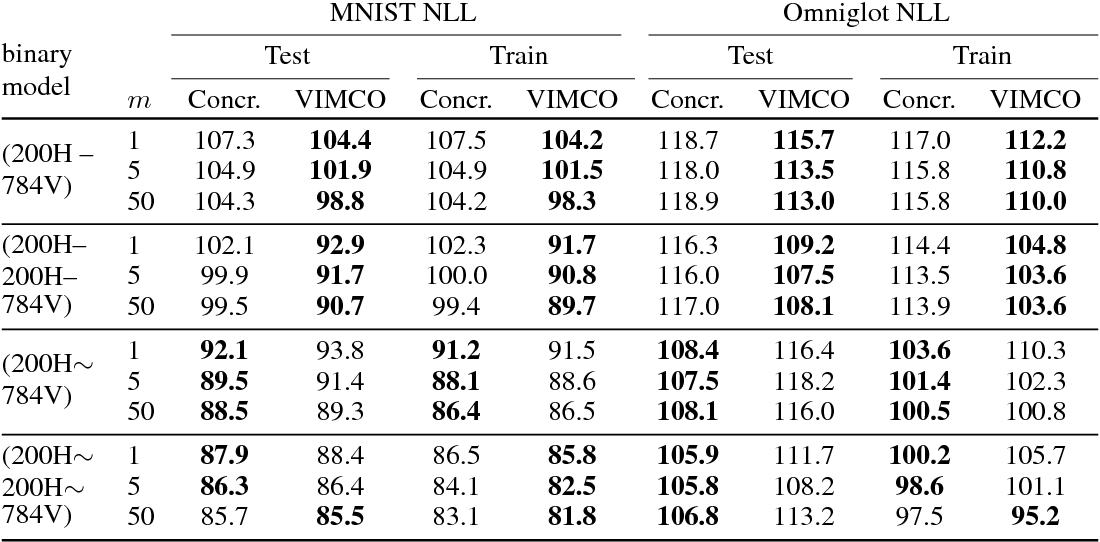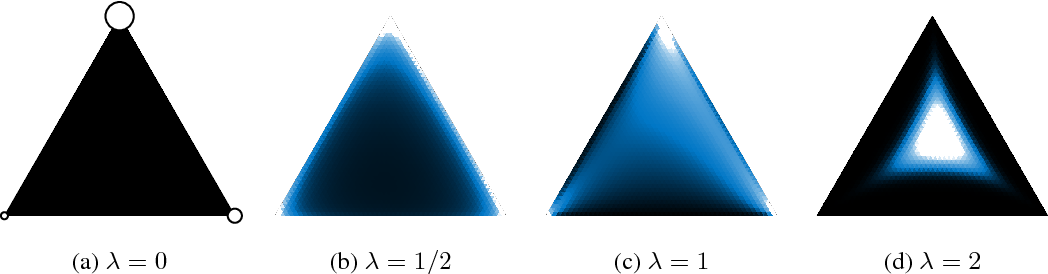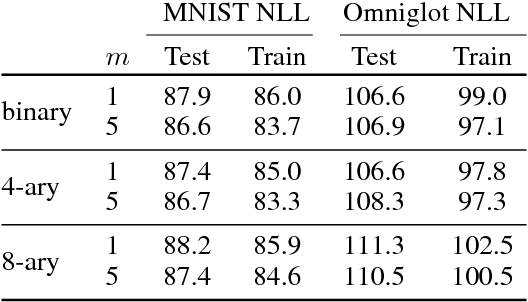The reparameterization trick enables optimizing large scale stochastic computation graphs via gradient descent. The essence of the trick is to refactor each stochastic node into a differentiable function of its parameters and a random variable with fixed distribution. After refactoring, the gradients of the loss propagated by the chain rule through the graph are low variance unbiased estimators of the gradients of the expected loss. While many continuous random variables have such reparameterizations, discrete random variables lack useful reparameterizations due to the discontinuous nature of discrete states. In this work we introduce Concrete random variables---continuous relaxations of discrete random variables. The Concrete distribution is a new family of distributions with closed form densities and a simple reparameterization. Whenever a discrete stochastic node of a computation graph can be refactored into a one-hot bit representation that is treated continuously, Concrete stochastic nodes can be used with automatic differentiation to produce low-variance biased gradients of objectives (including objectives that depend on the log-probability of latent stochastic nodes) on the corresponding discrete graph. We demonstrate the effectiveness of Concrete relaxations on density estimation and structured prediction tasks using neural networks.

Click to Read Paper and Get Code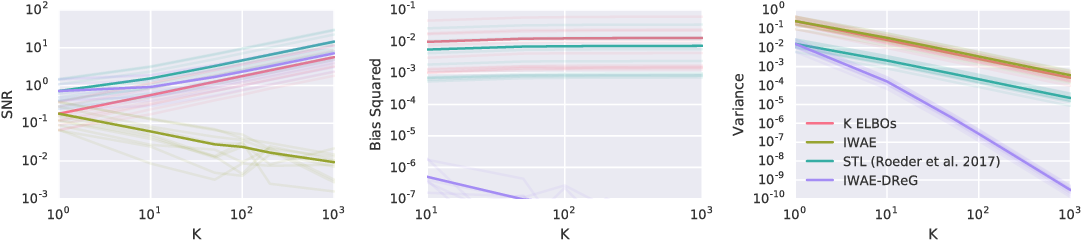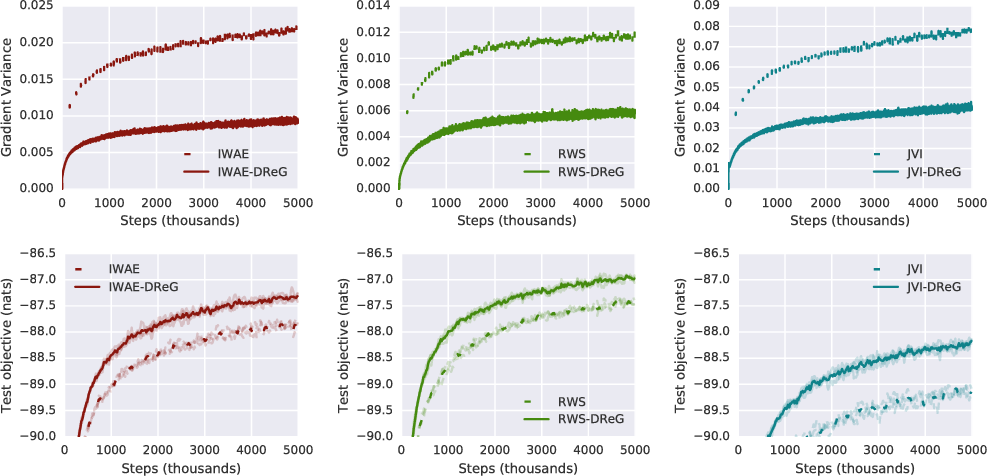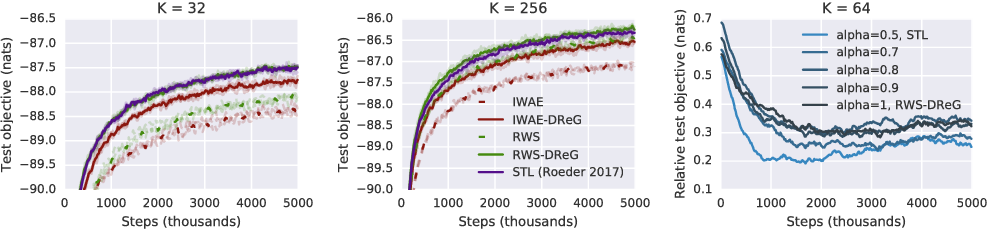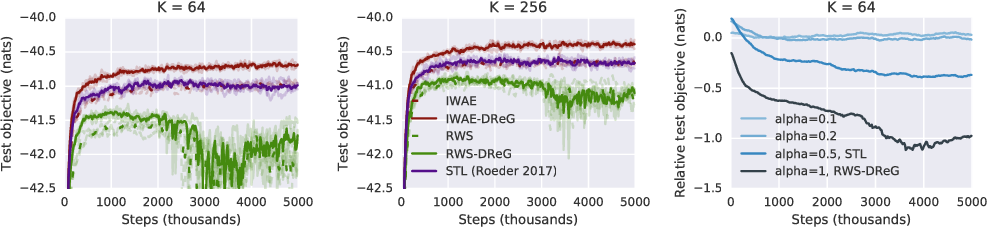Deep latent variable models have become a popular model choice due to the scalable learning algorithms introduced by (Kingma & Welling, 2013; Rezende et al., 2014). These approaches maximize a variational lower bound on the intractable log likelihood of the observed data. Burda et al. (2015) introduced a multi-sample variational bound, IWAE, that is at least as tight as the standard variational lower bound and becomes increasingly tight as the number of samples increases. Counterintuitively, the typical inference network gradient estimator for the IWAE bound performs poorly as the number of samples increases (Rainforth et al., 2018; Le et al., 2018). Roeder et al. (2017) propose an improved gradient estimator, however, are unable to show it is unbiased. We show that it is in fact biased and that the bias can be estimated efficiently with a second application of the reparameterization trick. The doubly reparameterized gradient (DReG) estimator does not suffer as the number of samples increases, resolving the previously raised issues. The same idea can be used to improve many recently introduced training techniques for latent variable models. In particular, we show that this estimator reduces the variance of the IWAE gradient, the reweighted wake-sleep update (RWS) (Bornschein & Bengio, 2014), and the jackknife variational inference (JVI) gradient (Nowozin, 2018). Finally, we show that this computationally efficient, unbiased drop-in gradient estimator translates to improved performance for all three objectives on several modeling tasks.

Click to Read Paper and Get Code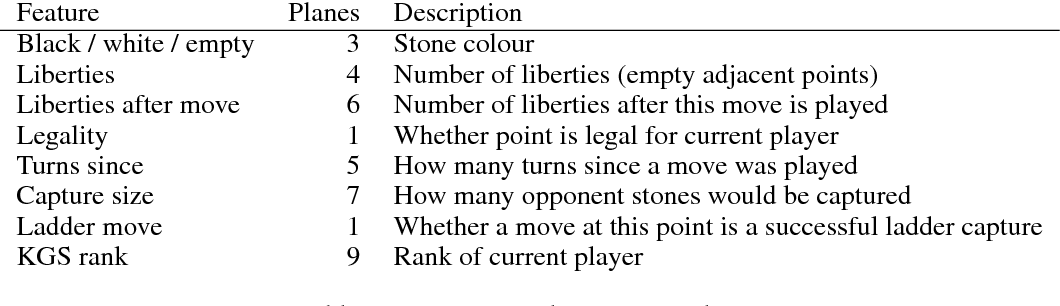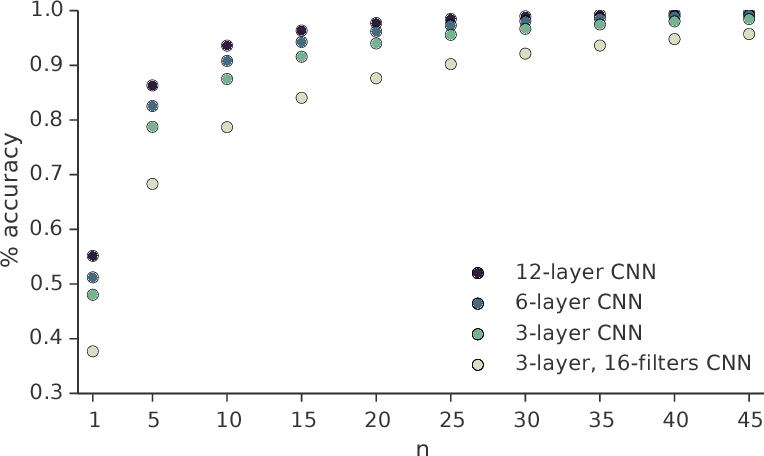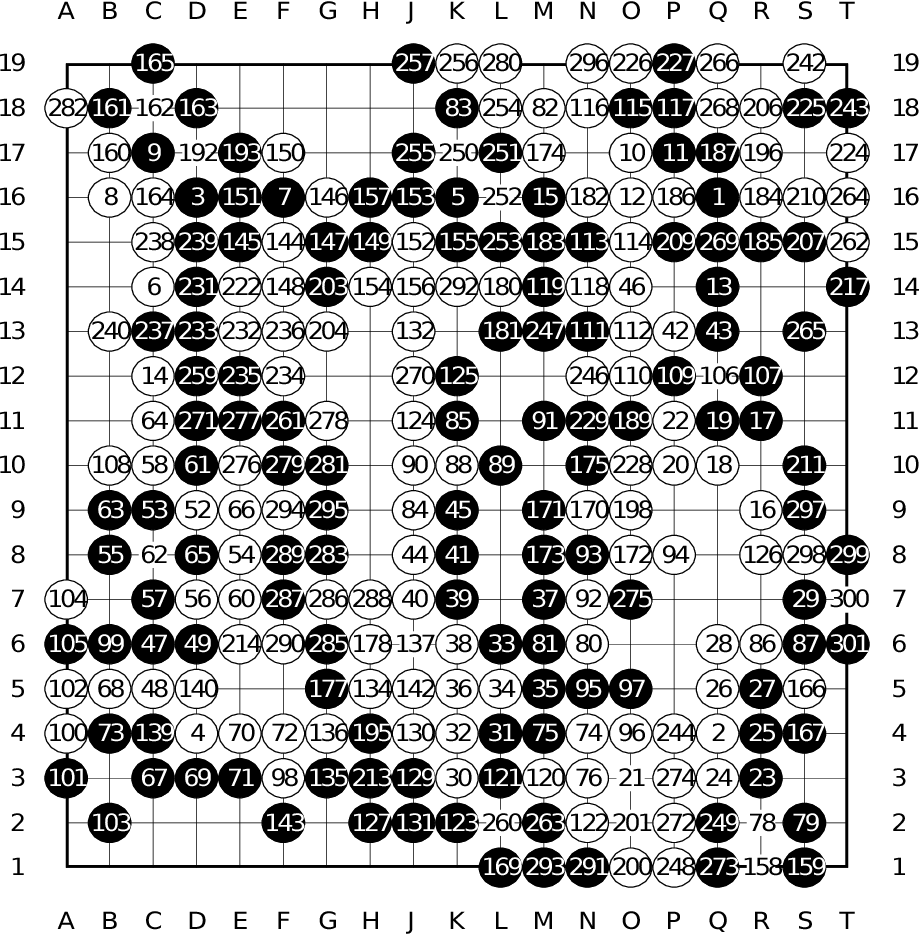The game of Go is more challenging than other board games, due to the difficulty of constructing a position or move evaluation function. In this paper we investigate whether deep convolutional networks can be used to directly represent and learn this knowledge. We train a large 12-layer convolutional neural network by supervised learning from a database of human professional games. The network correctly predicts the expert move in 55% of positions, equalling the accuracy of a 6 dan human player. When the trained convolutional network was used directly to play games of Go, without any search, it beat the traditional search program GnuGo in 97% of games, and matched the performance of a state-of-the-art Monte-Carlo tree search that simulates a million positions per move.

* Minor edits and included captures in Figure 2
Click to Read Paper and Get Code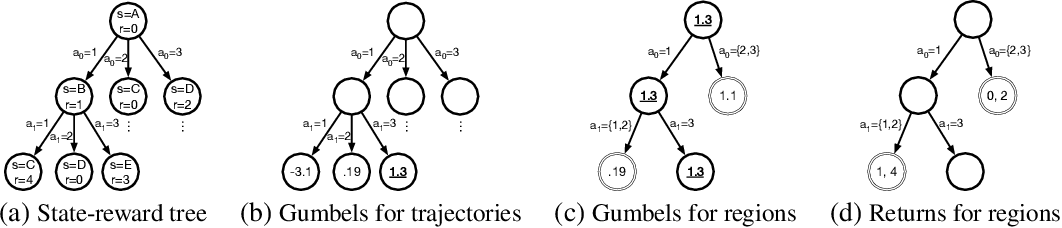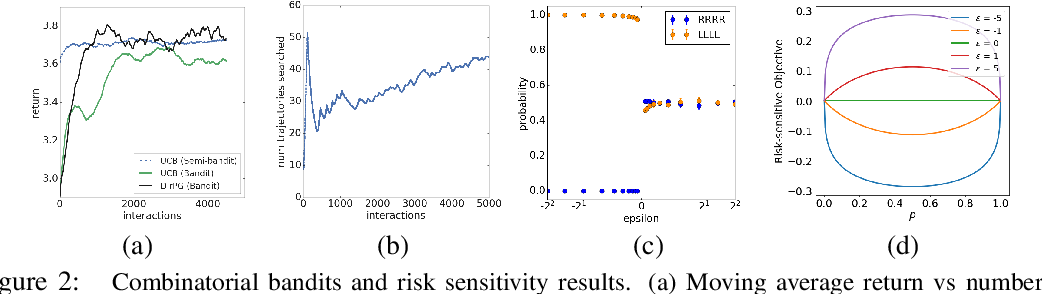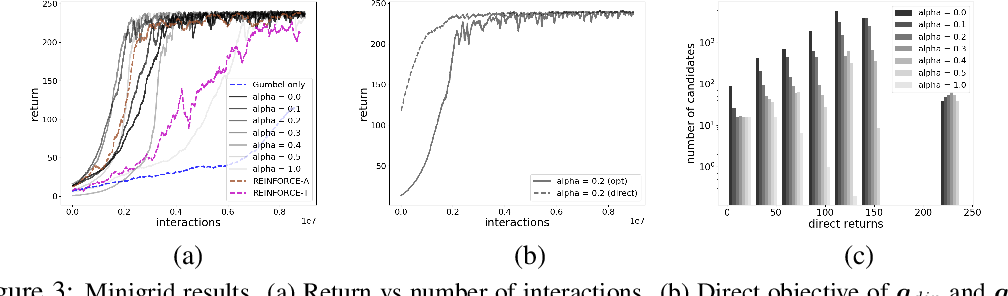Direct optimization is an appealing approach to differentiating through discrete quantities. Rather than relying on REINFORCE or continuous relaxations of discrete structures, it uses optimization in discrete space to compute gradients through a discrete argmax operation. In this paper, we develop reinforcement learning algorithms that use direct optimization to compute gradients of the expected return in environments with discrete actions. We call the resulting algorithms "direct policy gradient" algorithms and investigate their properties, showing that there is a built-in variance reduction technique and that a parameter that was previously viewed as a numerical approximation can be interpreted as controlling risk sensitivity. We also tackle challenges in algorithm design, leveraging ideas from A$^\star$ Sampling to develop a practical algorithm. Empirically, we show that the algorithm performs well in illustrative domains, and that it can make use of domain knowledge about upper bounds on return-to-go to speed up training.

Click to Read Paper and Get Code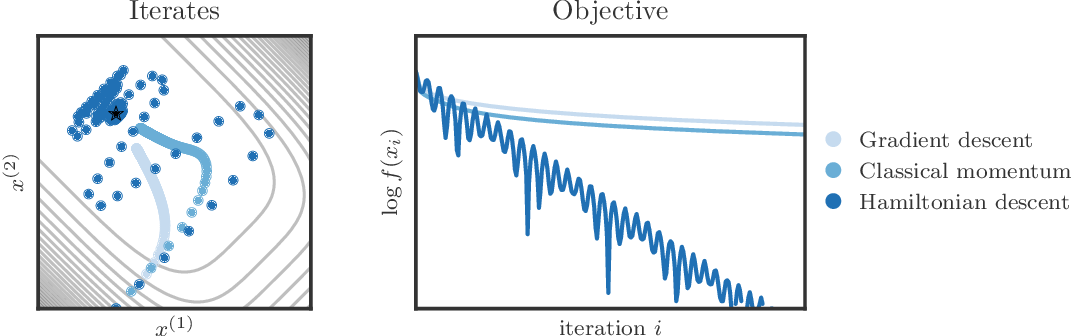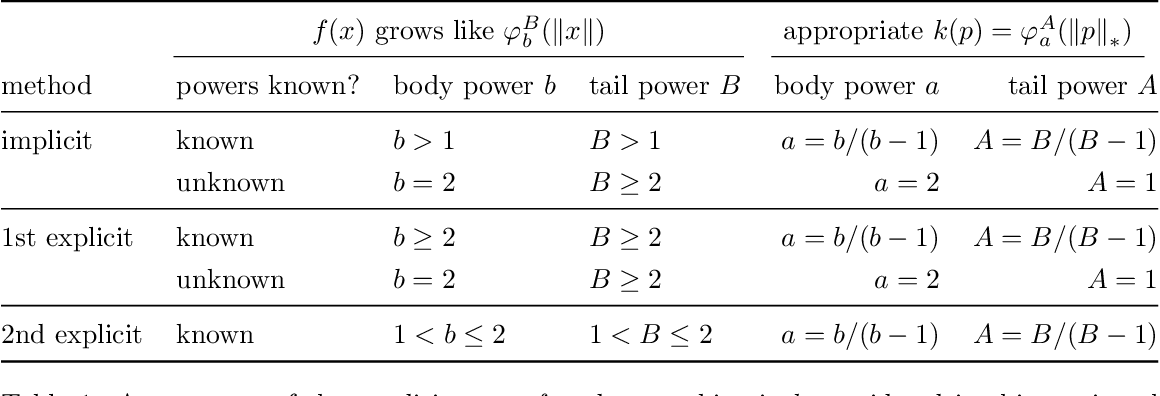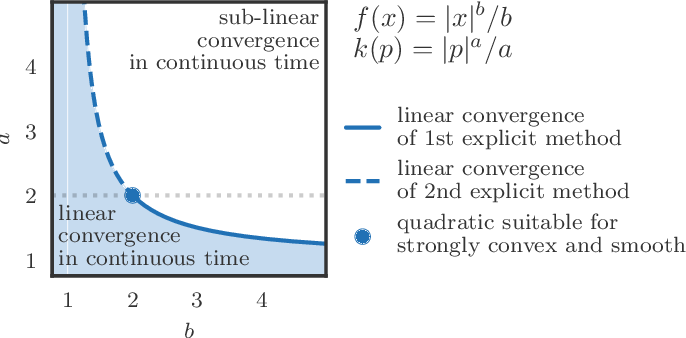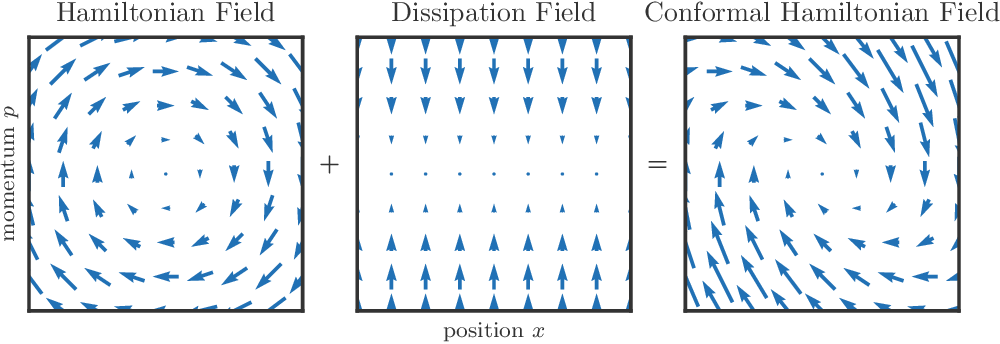We propose a family of optimization methods that achieve linear convergence using first-order gradient information and constant step sizes on a class of convex functions much larger than the smooth and strongly convex ones. This larger class includes functions whose second derivatives may be singular or unbounded at their minima. Our methods are discretizations of conformal Hamiltonian dynamics, which generalize the classical momentum method to model the motion of a particle with non-standard kinetic energy exposed to a dissipative force and the gradient field of the function of interest. They are first-order in the sense that they require only gradient computation. Yet, crucially the kinetic gradient map can be designed to incorporate information about the convex conjugate in a fashion that allows for linear convergence on convex functions that may be non-smooth or non-strongly convex. We study in detail one implicit and two explicit methods. For one explicit method, we provide conditions under which it converges to stationary points of non-convex functions. For all, we provide conditions on the convex function and kinetic energy pair that guarantee linear convergence, and show that these conditions can be satisfied by functions with power growth. In sum, these methods expand the class of convex functions on which linear convergence is possible with first-order computation.

Click to Read Paper and Get Code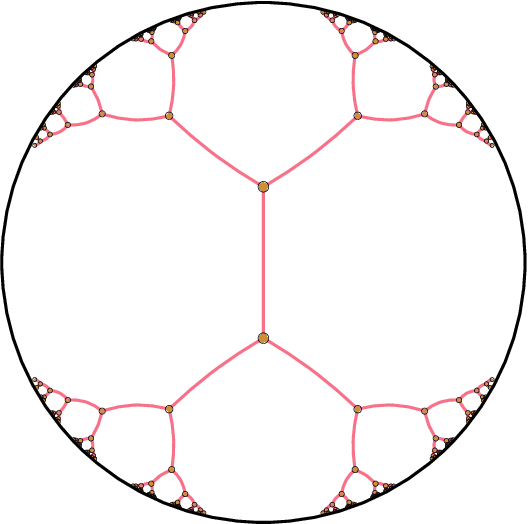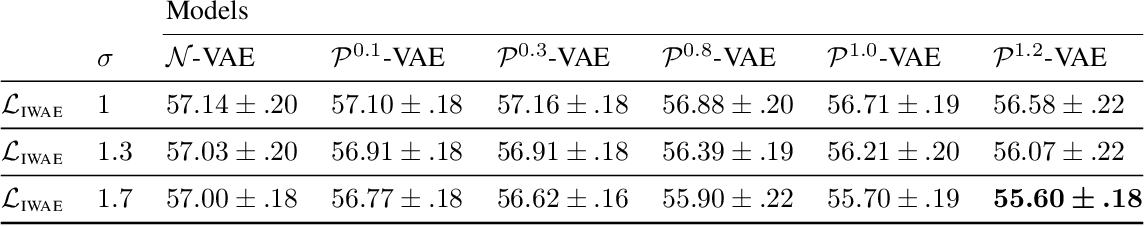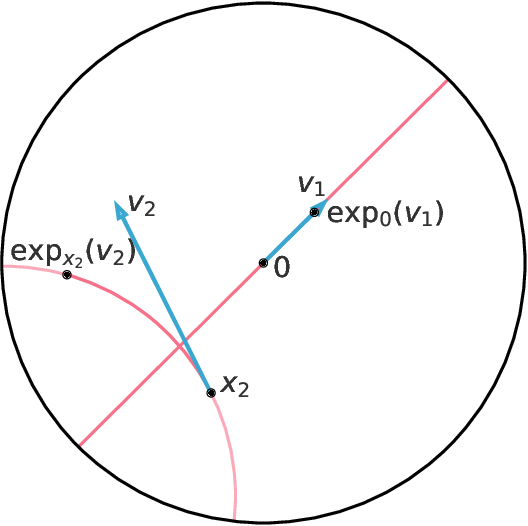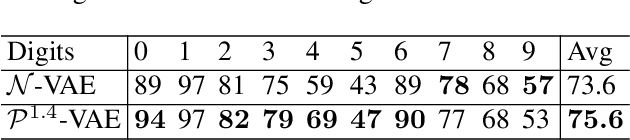The Variational Auto-Encoder (VAE) model has become widely popular as a way to learn at once a generative model and embeddings for observations living in a high-dimensional space. In the real world, many such observations may be assumed to be hierarchically structured, such as living organisms data which are related through the evolutionary tree. Also, it has been theoretically and empirically shown that data with hierarchical structure can efficiently be embedded in hyperbolic spaces. We therefore endow the VAE with a hyperbolic geometry and empirically show that it can better generalise to unseen data than its Euclidean counterpart, and can qualitatively recover the hierarchical structure.

Click to Read Paper and Get Code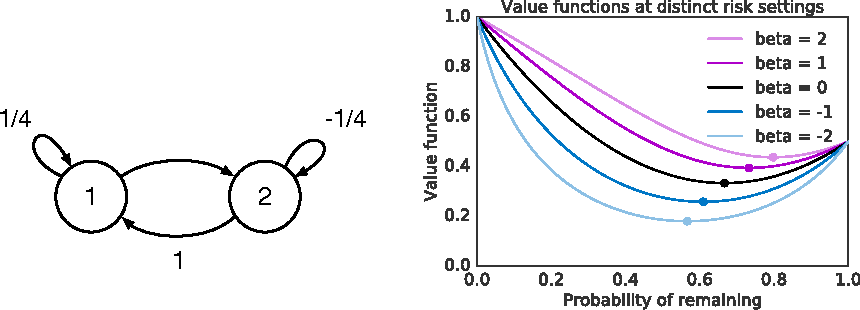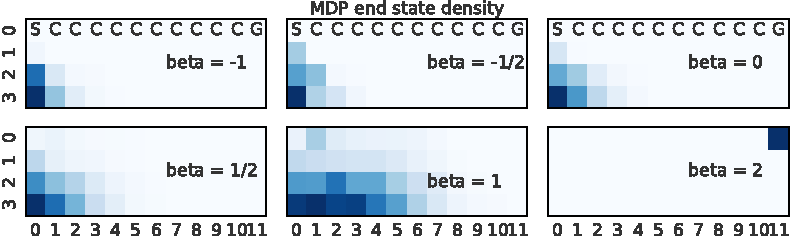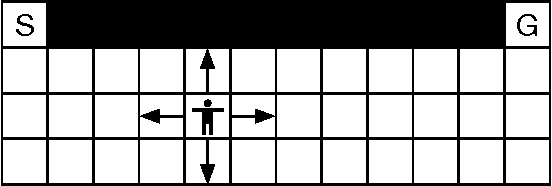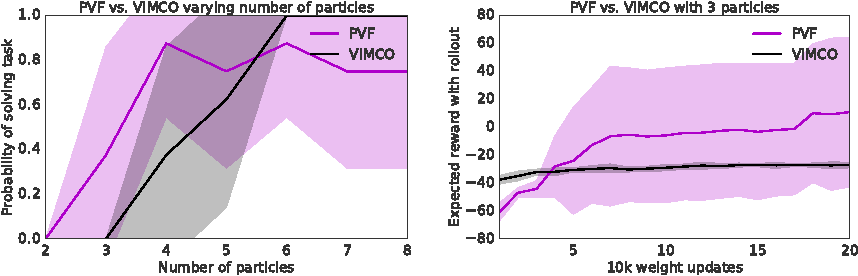The policy gradients of the expected return objective can react slowly to rare rewards. Yet, in some cases agents may wish to emphasize the low or high returns regardless of their probability. Borrowing from the economics and control literature, we review the risk-sensitive value function that arises from an exponential utility and illustrate its effects on an example. This risk-sensitive value function is not always applicable to reinforcement learning problems, so we introduce the particle value function defined by a particle filter over the distributions of an agent's experience, which bounds the risk-sensitive one. We illustrate the benefit of the policy gradients of this objective in Cliffworld.

Click to Read Paper and Get Code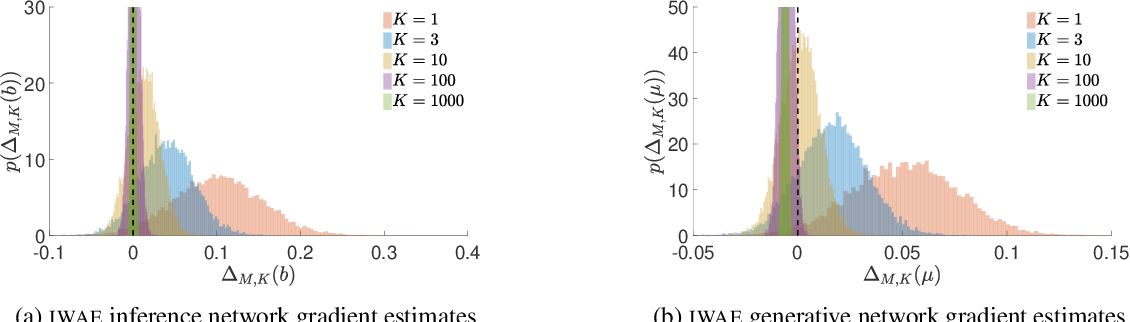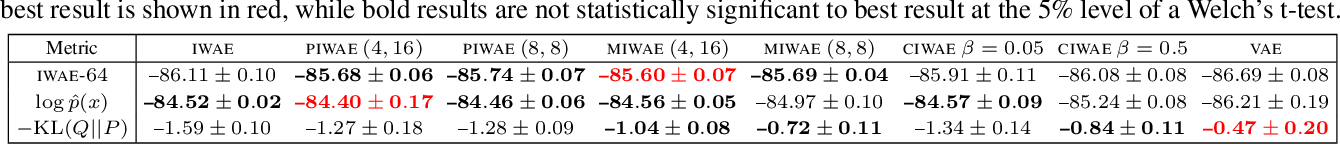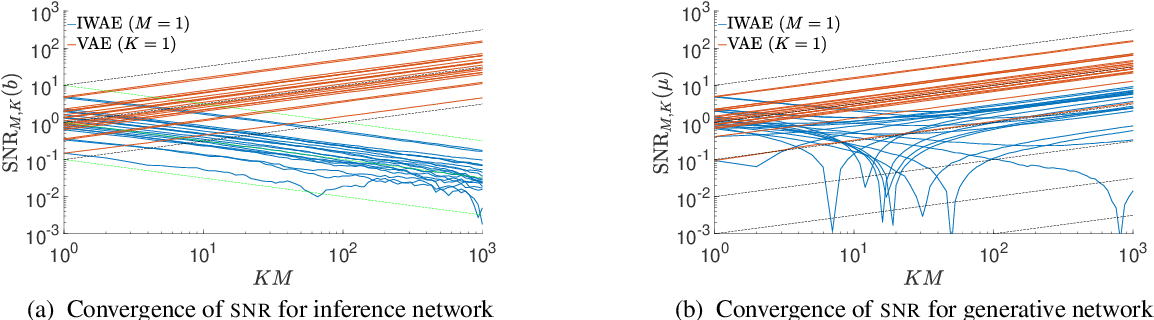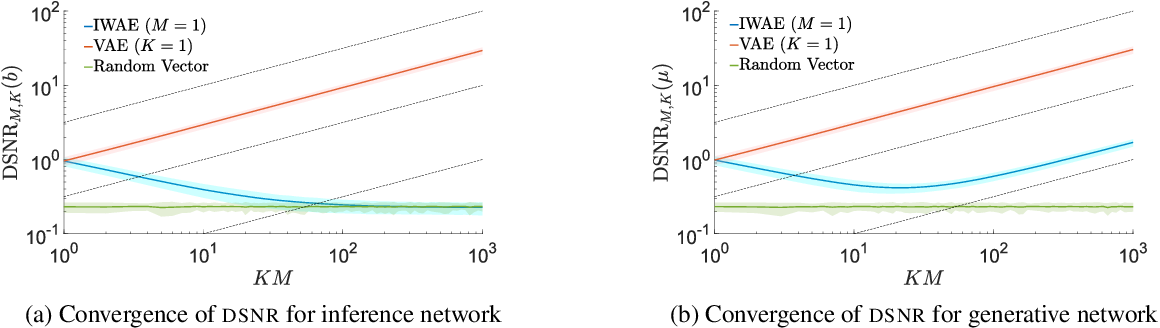We provide theoretical and empirical evidence that using tighter evidence lower bounds (ELBOs) can be detrimental to the process of learning an inference network by reducing the signal-to-noise ratio of the gradient estimator. Our results call into question common implicit assumptions that tighter ELBOs are better variational objectives for simultaneous model learning and inference amortization schemes. Based on our insights, we introduce three new algorithms: the partially importance weighted auto-encoder (PIWAE), the multiply importance weighted auto-encoder (MIWAE), and the combination importance weighted auto-encoder (CIWAE), each of which includes the standard importance weighted auto-encoder (IWAE) as a special case. We show that each can deliver improvements over IWAE, even when performance is measured by the IWAE target itself. Furthermore, our results suggest that PIWAE may be able to deliver simultaneous improvements in the training of both the inference and generative networks.

* To appear at ICML 2018
Click to Read Paper and Get Code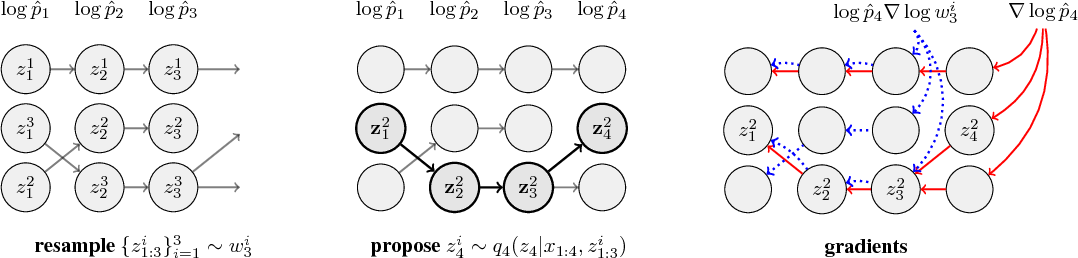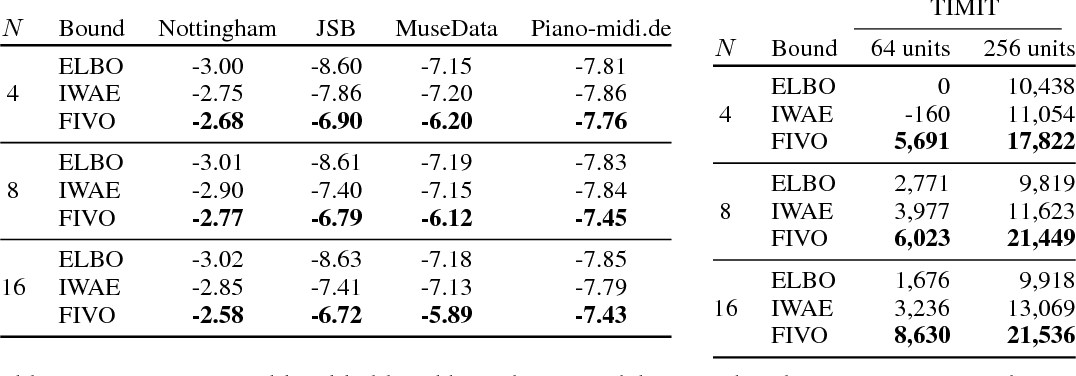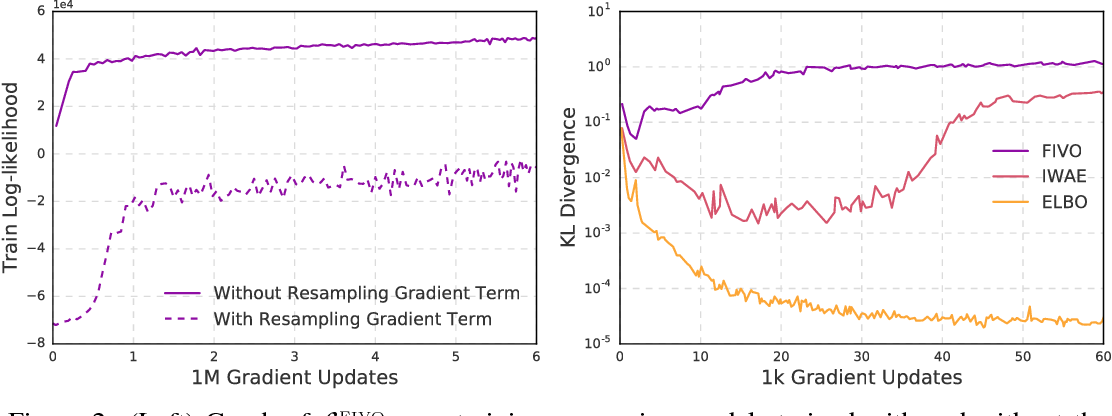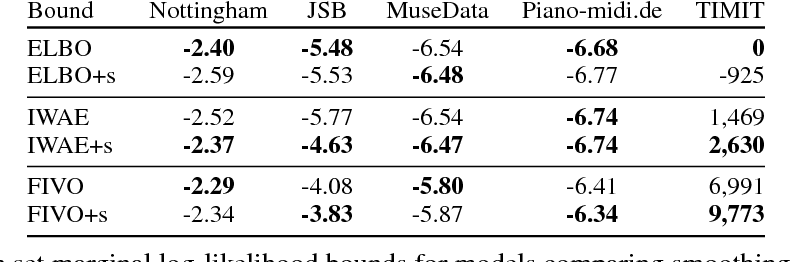When used as a surrogate objective for maximum likelihood estimation in latent variable models, the evidence lower bound (ELBO) produces state-of-the-art results. Inspired by this, we consider the extension of the ELBO to a family of lower bounds defined by a particle filter's estimator of the marginal likelihood, the filtering variational objectives (FIVOs). FIVOs take the same arguments as the ELBO, but can exploit a model's sequential structure to form tighter bounds. We present results that relate the tightness of FIVO's bound to the variance of the particle filter's estimator by considering the generic case of bounds defined as log-transformed likelihood estimators. Experimentally, we show that training with FIVO results in substantial improvements over training the same model architecture with the ELBO on sequential data.

Click to Read Paper and Get Code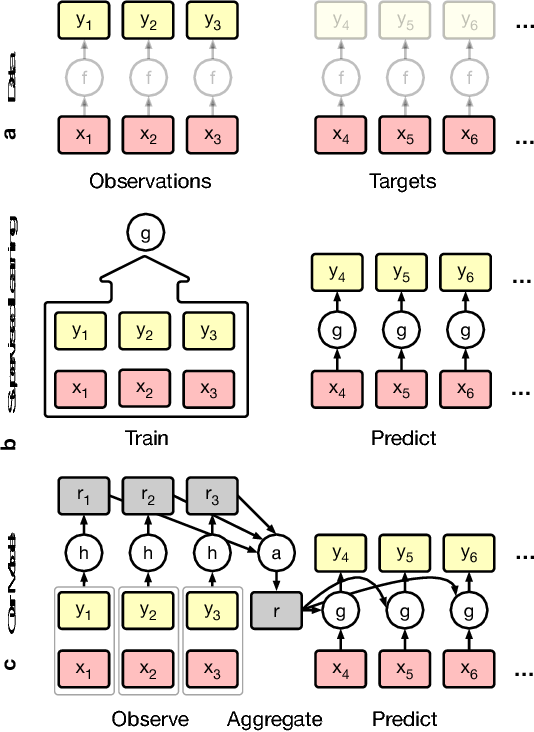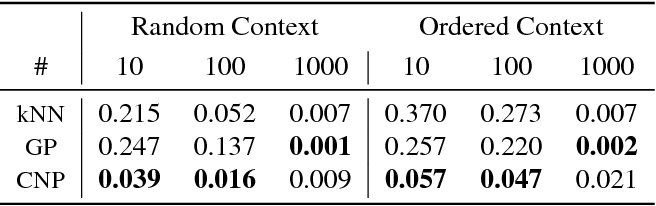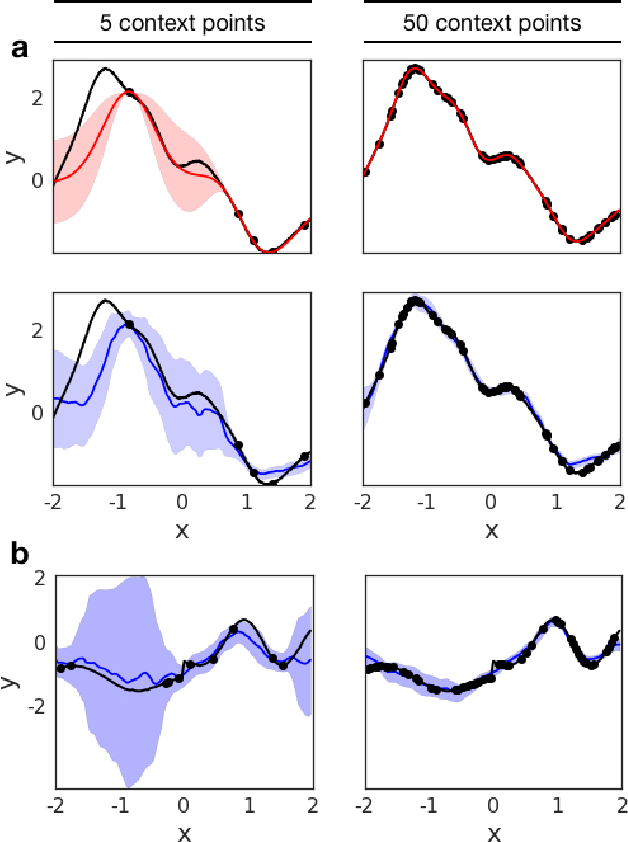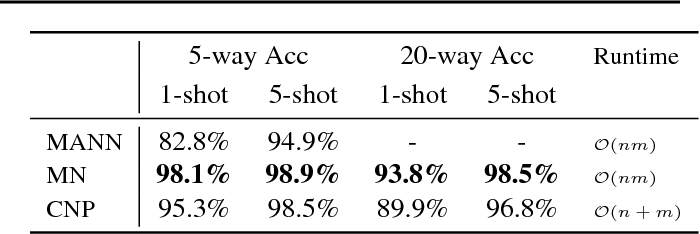Deep neural networks excel at function approximation, yet they are typically trained from scratch for each new function. On the other hand, Bayesian methods, such as Gaussian Processes (GPs), exploit prior knowledge to quickly infer the shape of a new function at test time. Yet GPs are computationally expensive, and it can be hard to design appropriate priors. In this paper we propose a family of neural models, Conditional Neural Processes (CNPs), that combine the benefits of both. CNPs are inspired by the flexibility of stochastic processes such as GPs, but are structured as neural networks and trained via gradient descent. CNPs make accurate predictions after observing only a handful of training data points, yet scale to complex functions and large datasets. We demonstrate the performance and versatility of the approach on a range of canonical machine learning tasks, including regression, classification and image completion.

Click to Read Paper and Get Code